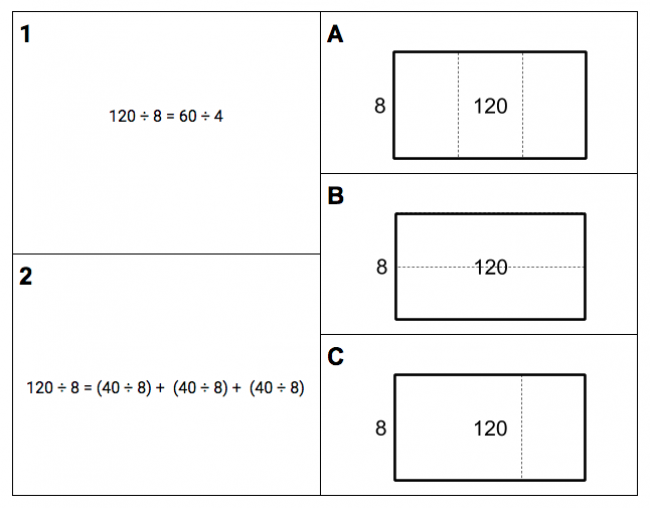# Division Area Models

Preview:This task draws students' attention to how division can be represented in an area model, and how each part of a division statement corresponds to the area model.

Connecting Representations
Big Idea:
Grade 4: Development of computational fluency and multiplicative thinking requires analysis of patterns and relations in multiplication and division.
Content:
understanding the relationships between multiplication and division, multiplication and addition, division and subtraction using flexible computation strategies (e.g., decomposing, distributive principle, commutative principle, repeated addition and repeated subtraction) using multiplication and division in real-life contexts and problem-based situations whole-class number talks
Curricular Competency:
Reasoning and analyzing: Develop mental math strategies and abilities to make sense of quantities
Understanding and solving: Develop, demonstrate, and apply mathematical understanding through play, inquiry, and problem solving
Understanding and solving: Visualize to explore mathematical concepts
Understanding and solving: Develop and use multiple strategies to engage in problem solving
Communicating and representing: Communicate mathematical thinking in many ways
Communicating and representing: Use mathematical vocabulary and language to contribute to mathematical discussions
Communicating and representing: Explain and justify mathematical ideas and decisions
Communicating and representing: Represent mathematical ideas in concrete, pictorial, and symbolic forms
Connecting and reflecting: Reflect on mathematical thinking